# Skeletonization using Proxies¶

In :
import numpy as np
import numpy.linalg as la
import matplotlib.pyplot as pt

import scipy.linalg.interpolative as sli

eps = 1e-7

In :
sources = np.random.rand(2, 200)
targets = np.random.rand(2, 200) + 3

pt.plot(sources, sources, "go")
pt.plot(targets, targets, "ro")

pt.xlim([-1, 5])
pt.ylim([-1, 5])

pt.gca().set_aspect("equal")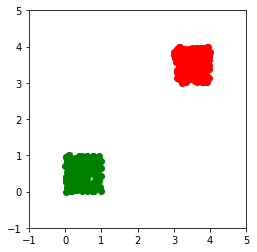In :
def interaction_mat(t, s):
all_distvecs = s.reshape(2, 1, -1) - t.reshape(2, -1, 1)
dists = np.sqrt(np.sum(all_distvecs**2, axis=0))
return np.log(dists)

In :
def numerical_rank(A, eps):
_, sigma, _ = la.svd(A)
return np.sum(sigma >= eps)


Check the interaction rank:

In :
numerical_rank(interaction_mat(targets, sources), eps)

Out:
9

Idea:

• Don't want to build whole matrix to find the few rows/columns that actually matter.
• Introduces "proxies" that stand in for
• all sources outside the targets or
• all targets outside these sources

## Target Skeletonization¶

In :
nproxies = 25

angles = np.linspace(0, 2*np.pi, nproxies)
target_proxies = 3.5 + 1.5 * np.array([np.cos(angles), np.sin(angles)])

In :
pt.plot(sources, sources, "go")
pt.plot(targets, targets, "ro")
pt.plot(target_proxies, target_proxies, "bo")

pt.xlim([-1, 5])
pt.ylim([-1, 5])

pt.gca().set_aspect("equal")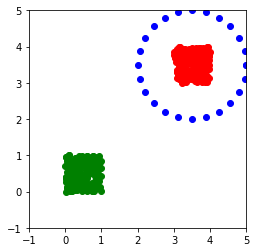Construct the interaction matrix from the target proxies to the targets as target_proxy_mat.

A note on terminology: The target_proxies are near the targets but stand in for far-away sources.

In :
target_proxy_mat = interaction_mat(targets, target_proxies)


Check its numerical rank and shape:

In :
numerical_rank(target_proxy_mat, eps)

Out:
24
In :
target_proxy_mat.shape

Out:
(200, 25)

Now compute an ID (row or column?):

In :
idx, proj = sli.interp_decomp(target_proxy_mat.T, nproxies)


Find the target skeleton as target_skeleton, i.e. the indices of the targets from which the remaining values can be recovered:

In :
target_skeleton = idx[:nproxies]


Check that the ID does what is promises:

In :
P = np.hstack([np.eye(nproxies), proj])[:,np.argsort(idx)]
tpm_approx = P.T @ target_proxy_mat[target_skeleton]

la.norm(tpm_approx - target_proxy_mat, 2)

Out:
6.738945834835623e-15

Plot the chosen "skeleton" and the proxies:

In :
pt.plot(sources, sources, "go")
pt.plot(targets, targets, "ro", alpha=0.05)
pt.plot(targets[0, target_skeleton], targets[1, target_skeleton], "ro")
pt.plot(target_proxies, target_proxies, "bo")

pt.xlim([-1, 5])
pt.ylim([-1, 5])

pt.gca().set_aspect("equal")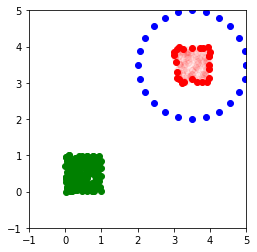What does this mean?

• We have now got a moral equivalent to a local expansion: The point values at the target skeleton points.
• Is it a coincidence that the skeleton points sit at the boundary of the target region?
• How many target proxies should we choose?
• Can cheaply recompute potential at any target from those few points.
• Have thus reduce LA-based evaluation cost to same as expansion-based cost.

Can we come up with an equivalent of a multipole expansion?

Check that this works for 'our' sources:

In :
imat_error = (
P.T.dot(interaction_mat(targets[:, target_skeleton], sources))
-
interaction_mat(targets, sources))

la.norm(imat_error, 2)

Out:
1.8776453951593488e-09

## Source Skeletonization¶

In :
nproxies = 25

angles = np.linspace(0, 2*np.pi, nproxies)
source_proxies = 0.5 + 1.5 * np.array([np.cos(angles), np.sin(angles)])

In :
pt.plot(sources, sources, "go")
pt.plot(targets, targets, "ro")
pt.plot(source_proxies, source_proxies, "bo")

pt.xlim([-1, 5])
pt.ylim([-1, 5])

pt.gca().set_aspect("equal")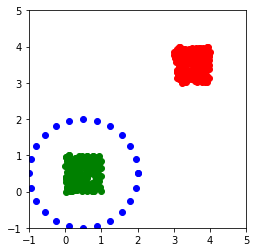Construct the interaction matrix from the sources to the source proxies as source_proxy_mat:

A note on terminology: The source_proxies are near the sources but stand in for far-away targets.

In :
source_proxy_mat = interaction_mat(source_proxies, sources)

In :
source_proxy_mat.shape

Out:
(25, 200)

Now compute an ID (row or column?):

In :
idx, proj = sli.interp_decomp(source_proxy_mat, nproxies)

In :
source_skeleton = idx[:nproxies]

In :
P = np.hstack([np.eye(nproxies), proj])[:,np.argsort(idx)]
tsm_approx = source_proxy_mat[:, source_skeleton].dot(P)

la.norm(tsm_approx - source_proxy_mat, 2)

Out:
5.2523961564651685e-15

Plot the chosen skeleton as well as the proxies:

In :
pt.plot(sources, sources, "go", alpha=0.05)
pt.plot(targets, targets, "ro")
pt.plot(sources[0, source_skeleton], sources[1, source_skeleton], "go")
pt.plot(source_proxies, source_proxies, "bo")

pt.xlim([-1, 5])
pt.ylim([-1, 5])

pt.gca().set_aspect("equal")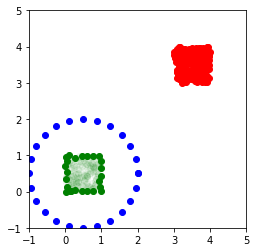Check that it works for 'our' targets:

In :
imat_error = (
interaction_mat(targets, sources[:, source_skeleton]) @ P
-
interaction_mat(targets, sources))

la.norm(imat_error, 2)

Out:
5.966880531704515e-09
• Sensibly, this is just the transpose of the target skeletonization process.
• For a given point cluster, the same skeleton can serve for target and source skeletonization!
• Computationally, starting from your original charges $x$, you accumulate 'new' charges $Px$ at the skeleton points and then only compute the interaction from the source skeleton to the targets.
In [ ]: